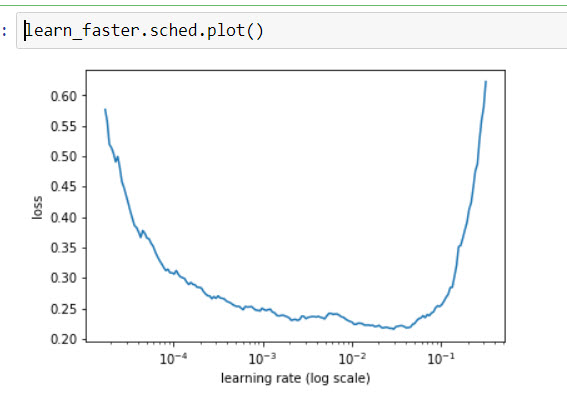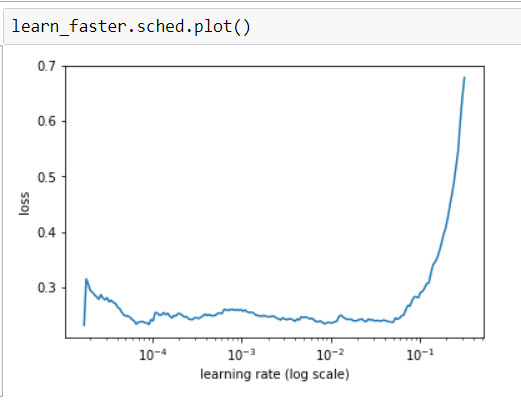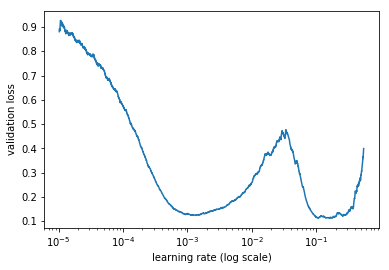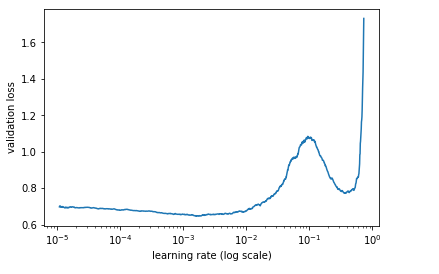Interpreting the `.sched.plot()` from `.lr_find()`

(Michael Slater) #1

For practice, I am analyzing the Dog Breed data.

I create a learner
learn_faster = ConvLearner.pretrained(arch, data, precompute=True)

Then I run the learning rate finder.
lrf=learn_faster.lr_find()

which gives me a learn_faster.sched.plot() result:

This shape makes sense – a learning rate of 0.05 to 0.01 is probably ok.

So I learn_faster.fit(0.01,2) to better fit the network.

For fun, I run the lrf=learn_faster.lr_find() again

The learn_faster.sched.plot() looks more bowl-likeNow it looks like the learning rate is more like 1e-4 or 1e-5, so I fit again: learn_faster.fit(1e-5,2)

Now the learn_faster.sched.plot() is almost flat.How I interpret this is this model has been .fit to the point that further fitting will make no improvement to the model. And if I choose aggressive fitting parameter (0.1+) then I’d just jump out of the minima I’m sitting in right now.

This corresponds with what I’m seeing on the accuracy results of each subsequent fit. The measurements never improved from the initial 92% I enjoyed on the first run, in fact worsened slightly as the fitting went on.

My question is: am I interpreting this chart correctly, or I am dong something stupid that is poisoning the results?

4 Likes

(Wira Dharma Kencana Putra) #2

Hi @karavshin,
Yes, your interpretation is heading in the right direction.
Something I would like to note is that it doesn’t have to be a local minima. Those sched.plot() you have are showing you that your model is in a “low gradient area”, such as, a local minima, a saddle point, or a plateau.

In this case, choosing aggressive parameter may or may not give you a better result though.
Either it will poison the result or not, it depends on the problem.

I hope it helps. Thanks.0 Likes

(Andrea de Luca) #3

Indeed.

0 Likes

(Arul Bharathi) #4

When I ran the lr_find() at first and then when I plotted learn.sched.plot(), I am getting the below graphWhat can we exactly interpret from a graph like this? Shall I call the first minima as the global minima? Also I have picked 1e-3 as the starting learning rate for this problem, is that right?

0 Likes

(Ujjwal Kumar) #5

I am also having a similar plot for earn.sched.plot()So should I go with lr=0.001 or something like lr=0.1
As there is a change that might be stuck in a plateau and higher learning rate might help me recover from it.

0 Likes